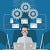# Citation: The content for this Article has been taken from OneFourthLabs on Deep Learning Course.

Source: Deep Learning on Medium

Citation: The content for this Article has been taken from OneFourthLabs on Deep Learning Course.

Artificial Intelligence is the collection of certain Tasks, Abilities and Methods just like humans have the ability to see, listen, talk, read, write & decision making. An AI system can have Computer Vision, Speech recognition, NLP, Planning/Decision making to complete one of these tasks using predefined or known methods such as Convolutional Neural Network, Deep Neural Network etc.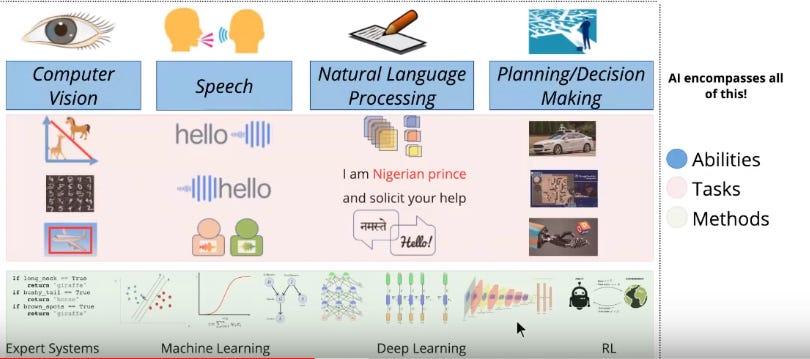Artificial Intelligence Overview

To develop an intelligent system or a machine to learn by itself, there is a set of requirements or pre-requisites which should be available/applicable.

There are mainly 6 elements of Machine Learning:

1. Data:

We have data everywhere like structured data, video data, text data etc. But only having random data doesn’t mean we can use it for machine learning but we need specific type of data. Say for example we have medical scanning report data, in which we have scan reports which is converted to numbers and we need to find out whether that report has some kind of abnormality/anomaly.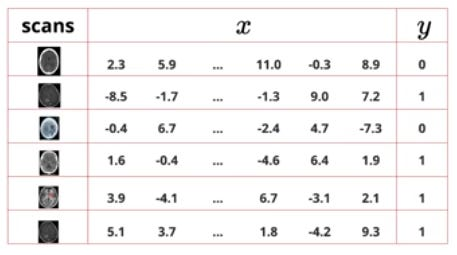Data in Numeric(x) and Label(y)

For making meaning out of the data we need to have examples of known results data meaning we should have reports with doctors judgement whether each of these reports have anomaly or not, so say x is the image input in numbers and y is judgement of the doctor. Feeding this data we can train a machine to learn the relation between data and output and for any new image we can classify it.

Image Data: The Data will be very high dimensional for each of the image data, considering the image of 30×30 pixel size, so the dimension of the image will be 900 real numbers.

Video Data: Again a video is a set of images which is defined by frames per second. A video can be broken down to images and then into pixels which can be represented by numbers, but the dimension will be huge for video.

Text Data: There are processing tools which can be used.

Speech Data: It can be represented by different frequencies, amplitude as numbers.

Any data format, has to be encoded to numerical format and its dimension will be too high.

Data Curation: We can find some test data from Google AI, data.gov.in, UCI machine learning repository. Please check it over there.

2. Tasks:

A task is nothing but a firm definition of what your input is and what your output should be. Once we have the data in various formats, like image, text reviews, ratings etc. we can define tasks.

For ex: Amazon Product Selling page has all kinds of information like image, description, technical details, reviews and FAQ’s

Many of the user’s questions can be answered from already available information on page, so we have to apply a function to all available information to get output

Y = f(X)

Here Y is the expected output and X are the various information like description, technical details, reviews, FAQ’s etc we have.

We can categorize tasks in various ways:

Supervised Tasks: In supervised setting, both x and y are important, x defines the image and y is the label for that image describing whether there is text in the image or not. Based on this we can define a classifier which learns the relation between x and y and ouput of the classifier is 1 or 0 depending on whether it has text or not.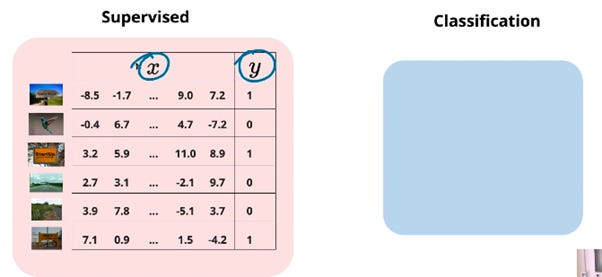Task Classification in Supervised Data

Regression: Here we are not only interested in knowing whether it has text or not but also want to know in what location exactly is the text located in image. With this we are finding some real values i.e. the coordinates in the box.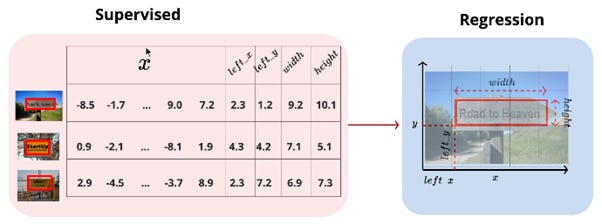Regression Classification in Supervised Task

UnSupervised Tasks: Given all the image data, we can use clustering to segregate all the images in different categories. We don’t know the y labels and we don’t know what each cluster means. The machine will try to form some patterns, but we need to build as per our requirement.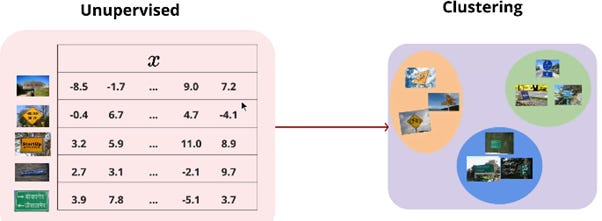Clustering in Unsupervised Task

Generation Task: Given some paintings images which form our x data, machine should build similar paintings. There is no y part.

3. Models:

Discovering a function that captures the relationship between y and x, basically we will have to find f(x) for y.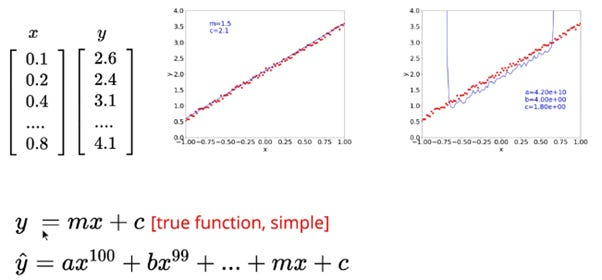Graph representation on linear solution

We can start with a simple linear function y = mx + c, this linear line should be passing through all the points on given data in graph. Then we can start with a polynomial function taking all the constants from given data. We can upgrade the degree of the polynomial until we get the line crossing closely or exactly on the points in graph.

An ML engineer’s job is to find one suitable function to solve the problem. But we should not directly try with a very complex function. Consider above example, the points on the graph are almost lying along the linear line, so in this case, if we use a very higher degree polynomial function, then all the coefficients except m and c will be zero but we are calculating them all and at end it comes to zero and we will be left with mx + c function.

4. Loss Function:

A loss function defines which model is better for our problem out of many selected models.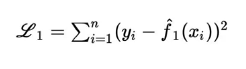Loss Function

A machine can try with different set of values to reach a feasible solution to the problem but the very exact solution is merely obtained. To know out of all the functions which one is suitable for our problem, we can apply loss functions on give true value and the calculated value of y. The lesser the loss means the more correct is our solution to the problem.

We have many loss functions, the above one is square error loss

Cross entropy loss

KL divergence

5. Learning Algorithm:

In Model forming we have tried a lot of functions with different set of parameters to reach a minimal loss corresponding to our true value. We cannot simply try with any set of parameters in our polynomial function. This can be defined as a search problem.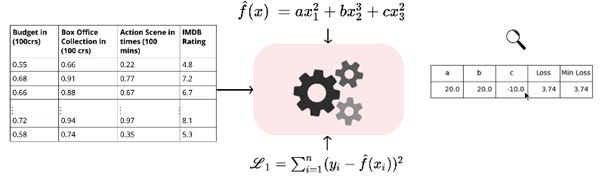Finding feasible Algorithm to a problem

But say for example, Here we can define a upper and lower limit say -20 to +20 (just any random) and we can apply brute force in above function to find minimal loss for these three values of a, b and c for given x values by incrementing it step by step. In real world it will be 1000’s of polynomials, and it will be not feasible to calculate and it will be too expensive to do so.

We need an efficient way of computing these parameters given the data, model and a loss function. Linear algebra and Calculus provide the base for Machine Learning Algorithm.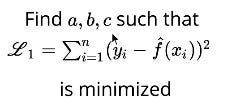minimize Loss

The functions provided are neural network family of functions such as Gradient Descent, Adagard, RMSProp, Adam. Apart from these Backpropagation is used in training deep neural networks.

6. Evaluation:

Evaluation is the score provided to the ML model

Suppose you are building an image classifier, we have true labels and we can compare these true labels with predicted labels and find out the accuracy of our model.

For instance, whether the output predicted by the model is same as true output.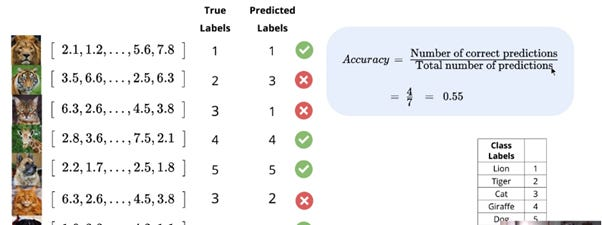Evaluation for Efficiency

Evaluation is different from the loss function because it exactly says out of 100 times only specific times my answer will be correct. This is needed for precision and efficiency in real world.